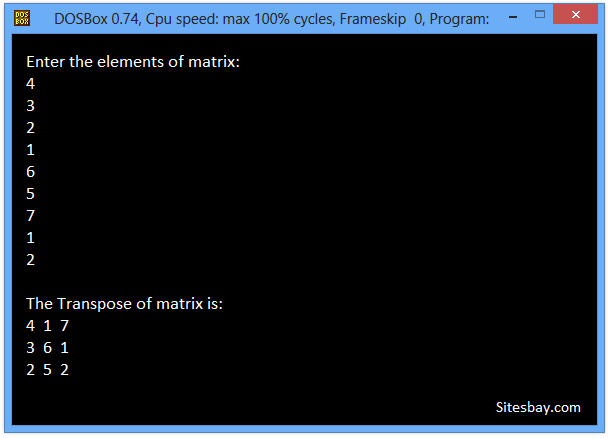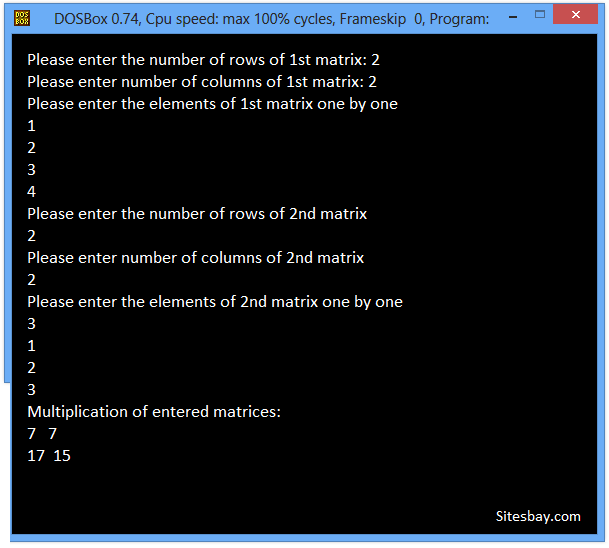# Transpose Matrix Program in C

## C Program to Transpose Matrix

To transpose any matrix in C Programming language, you have to first ask to the user to enter the matrix and replace row by column and column by row to transpose that matrix, then display the transpose of the matrix on the screen as shown here in the following C program.

### Examples to C Program to Transpose Matrix

The transpose of this matrix is shown below: Rows and columns are interchanged, rows of original matrix becomes column in transpose and columns of original matrix becomes rows in transpose.

## Example

```----------------
| 1  | 2  | 3  |
| 4  | 5  | 6  |
| 7  | 8  | 9  |
| 10 | 11 | 12 |
----------------
```

## Example

```---------------------
| 1  | 4  | 7  | 10 |
| 2  | 5  | 8  | 11 |
| 3  | 6  | 9  | 12 |
---------------------
```

To understand below example, you have must knowledge of following C programming topics; String in C, for looping statements we need know For Loop in C and Array in C.

## C Program to Transpose Matrix

```#include<stdio.h>
#include<conio.h>

void main()
{
int a,b,i,j;
clrscr();
printf("\nEnter the elements of matrix: ");
for(i=0;i<3;i++)
{
for(j=0;j<3;j++)
{
scanf("%d",&a[i][j]);
}
}
printf("\nThe Transpose of matrix is:\n");
for(i=0;i<3;i++)
{
for(j=0;j<3;j++)
{
b[i][j]=a[j][i];
printf("\t%d\t",b[i][j]);
}
printf("\n");
}
getch();
}
```

## Output

```Enter the elements of matrix:
4
3
2
1
6
5
7
1
2

The Transpose of matrix is:
4  1  7
3  6  1
2  5  2
```## C Program to Transpose Matrix

```#include<stdio.h>
#include<conio.h>

void main()
{
int m, n, p, q, c, d, k, sum = 0;
int m1, m2, m3;
clrscr();
printf("Please enter the number of rows of 1st matrix: ");
scanf("%d", &m);
printf("Please enter number of columns of 1st matrix: ");
scanf("%d", &n);
printf("Please enter the elements of 1st matrix one by one\n");
for (  c = 0 ; c < m ; c++ )
{
for ( d = 0 ; d < n ; d++ )
{
scanf("%d", &m1[c][d]);
}
}
printf("Please enter the number of rows of 2nd matrix\n");
scanf("%d", &p);
printf("Please enter number of columns of 2nd matrix\n");
scanf("%d", &q);
if ( n != p )
{
printf("The entered matrices can�t be multiplied each other.\n");
printf("To multiply two matrices of X and Y, the number of columns in X must be equal to the number of rows in Y");
}
else
{
printf("Please enter the elements of 2nd matrix one by one\n");
for ( c = 0 ; c < p ; c++ )
for ( d = 0 ; d < q ; d++ )
scanf("%d", &m2[c][d]);
for ( c = 0 ; c < m ; c++ )
{
for ( d = 0 ; d < q ; d++ )
{
for ( k = 0 ; k < p ; k++ )
{
sum = sum + m1[c][k]*m2[k][d];
}
m3[c][d] = sum;
sum = 0;
}
}
printf("Multiplication of entered matrices:\n");
for ( c = 0 ; c < m ; c++ )
{
for ( d = 0 ; d < q ; d++ )
printf("%d\t", m3[c][d]);
printf("\n");
}
}
getch();
}
```

## Output

```Please enter the number of rows of 1st matrix: 2
Please enter number of columns of 1st matrix: 2
Please enter the elements of 1st matrix one by one
1
2
3
4
Please enter the number of rows of 2nd matrix
2
Please enter number of columns of 2nd matrix
2
Please enter the elements of 2nd matrix one by one
3
1
2
3
Multiplication of entered matrices:
7   7
17  15
```WIFI CCTV Camera